The first thing to understand is that a lsystem has its own orientation in 3D space, the symbols are
explaned in the Lparser.txt file.
^
+   |   -
|
-----------------
The arrow points at the "draw direction", to move left: use +, to move right: use -
An example: ( text after the "#" sign is ignored by the Lparser )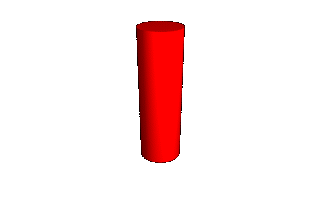2     # recursion depth
25    # angle
50    # thickness as % of length
F     # draw a full length
@     # end of file mark

If you view the result of file 1 (I always mean: viewing with the Lviewer), you just see a red bar standing up
straigth as in this picture....
Now try the next file:2
25
50
+F
@

Viewing the result shows the same red bar, but now tilted in an angle of 25 degrees. Watching the picture
you see that the object is tilted somewhat towards the screen, this is because the Lviewer shows the
object from behind (default). So "+F" in this case means "turn left by the angle and draw a full length", "-F"
would mean "turn right by the angle and draw a full length". Remember we defined the angle to be 25
degrees. Try the file with different angle and thickness settings, so you get used to it.
Now the recursion depth: recursion is a loop, the file loops in itself as many times as defined.
An example:4
25
50
A # the axiom
A = +FA
@

This file will loop 4 times, lets do that without the Lparser, just on paper:
loop 1: +F       gives A = +F
loop 2: +F+F     gives A = +F+F
loop 3: +F+F+F   gives A = +F+F+F
loop 4: +F+F+F+F gives A = +F+F+F+F
In every loop the A gets an "+F" added because we defined A to become +FA. Try to imagine what object
this file should generate. First an angle of 25 deg, followed by a full length, again an angle change of 25
deg and again a full length and so forth. If you repeate this long enough it should become a cirkel. Try the
file with the Lviewer..you'll see an open ended circle.
We also have the "-" option to try out, so: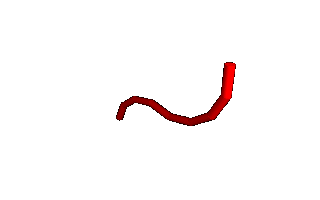4
25
50
AB
A = +FA
B = -FB
@

Reading this file tells us (as final string): +F+F+F+F-F-F-F-F (which is equal to AB). Again: try to imagine
what this object would look like, than try it with the Lviewer.
As you probably noticed the Lparser draws every next F ON the previous one, this is because we just
typed "AB"; so B follows A. We can alter the definition in such a way that this will not happen, we use the
"[ ]" symbols for this. Everything between the "[ ]" symbols is drawn but not counted for.. i.e. the Lparser
draws but does not remember where it left off between the brackets, it remembers only where it left off
before the brackets.
A file to explain this:4
25
50
[A][B]
A = +FA
B = +FB
@

The final string will be: [+F+F+F+F][-F-F-F-F] So the Lparser starts at the bottom, draws the fist string [A],
than again starting from the bottom it draws the second string [B]. We can make this as complex as we
want, but do not randomly try things in the files without knowing what you are doing, its fun OK, but you will
learn little from it.
"+" and "-" is left and right (around up vector:), but there are more orientation commands, try this: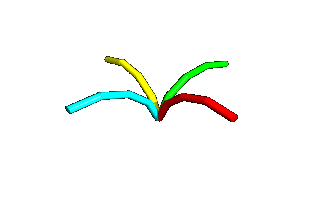4
25
50
[A]c[B]c[C]c[D]
A=+FA
B=-FB
C=^FC
D=&FD
@

The final string will be: [+F+F+F+F][-F-F-F-F][^F^F^F^F][&F&F&F&F]. The Lparser.txt teaches us that "^"
and "&" are pitching up and down around the left vector. So this should be left and right on the 3D axis in
the space around the object. Also "c" is added in the final definition, this is the color command, every time
it is used the color changes. By editing them out one by one it becomes clear which definition becomes
what object in the drawing.
View the file, and try deeper recursions. What happens if the recursion gets above 14 ? What happens if
the "c" command is in between the brackets instead outside them? Why?
Notice that +F in these files means "left 25 degrees, draw F", it can also be defined as +(25)F. That way
you can use angles different from the predefined angle.
"E" (after the lparser has gone through all the loops!) can be inserted into the the form itself, imagine what
that would do: placing the form on each of its own branches!
try this file: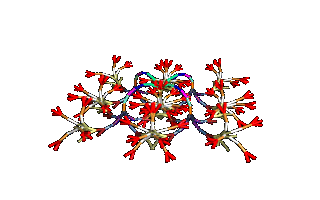8
25
50
E
E = [A][B][C][D]
A = c+FAE
B = c-FBE
C = c^FCE
D = c&FDE
@

As you can see: "E" is inserted onto every branch. This is a fractal form, i.e. a form forever repeating itself
in itself. There are some differences, we pre-defined the first form, but hey... the same thing goes for the
famous snowflake fractal! Lets do that Snowflake as well! By now you will know what is done in the first
steps creating a .ls file setup:
Lets do this by the book: "The construction of the Koch curve proceeds in stages. In each stage the
number of segments increases by a factor of four." The initiator (the smallest form of the fractal) is "F"
of course, the generator (the smallest fractal part still having the form of the fractal) is a line like: _/\_
The angles are clearly 45 degrees, so the generator should be defined as: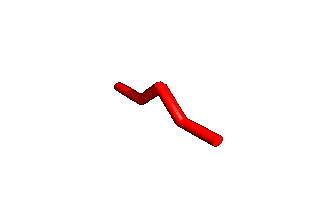4   # no need for deep recursion yet.
45  # the angle of 45 degrees.
50  # well... 50 is a good thickness.
A   # the axiom (for now).
A = ++F-F++F-F--
@

So, that was simple, now it is getting a bit more complex: We just made the generator, this is the form that
must be repeated in itself, keeping the same form. Now this generator is used to create the same form
again: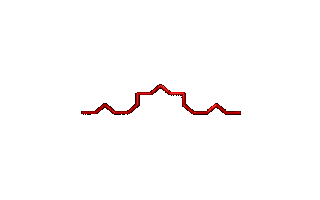4
45
50
B
A = ++F-F++F-F--
B = A-A++A-A
@

As you see, the generator is used to create the same form as the generator itself, the beginning of the
snowflake fractal is made. We could get carried away and define C D E (not F!) G etc. every time
including the growing form....
like: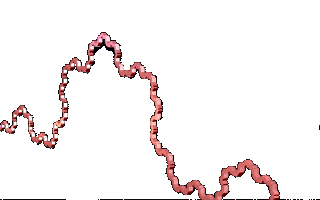4
45
50
D
A = ++F-F++F-F--
B = A-A++A-A
C = B-B++B-B
D = C-C++C-C
@

If you view this file the snowflake KOCH fractal is there! And without recursion, we defined every bit of the
form. That is not the way. Assume you want it to be repeated 100 times...some typing to do!, not even
speaking of the symbols you have to use to define them all, the alfabet (with use of upper and lower
case letters) is to short to define al the forms!
So we have to use recursion depth, like this:4
45
50
C
A = ++F-F++F-F--
B = A-A++A-AC
C = cB-B++B-B
@

As you read the file, you will see that the "C" form is included into the "B" form, which itself is part of the
"C" form, etc. etc. Now we can use recursion depth! Try it, but keep the level below 12 :-)
Every new "C" form has a new color, defined with the c at the beginning of the C definition.
Maybe it is a good idea to make another fractal-like object. It's a simple one, called the Pythagoras tree. It
starts with the mean branch, then on it, under an angle of 45 degrees, two branches with a length of
1/SQR(2) times the mean branch. On them again two branches under 45 degrees with a length of
1/SQR(2) times the PREVIOUS length and so on, all in the same plane... Starting from the base form (F)
and working our
way up:5
45
10
F
@

This is the mean branch, now we have to make the two branches on it, with a length of 1/SQR(2) the mean
branch, we use the command '(0.7071) for it.
Using this the file should look like this: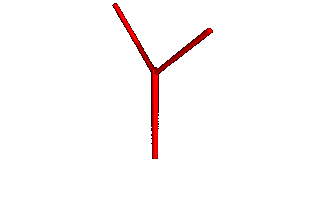5
45
10
A
A = F[+'(0.7071)F][-'(0.7071)F]
@

As you see, I used the branching commands [ ] to do the job. Now the only thing that remains is: get it
recursive! Calculating the lengths is a rule like 0.7071*0.7071*0.7071.....etc, each recursion the length is
decreased by the same factor, this is the first thing that could be made recursive.
Doing just that, we get:5
45
10
A
A = F[+BF][-BF]
B = '(0.7071)
@

B is now incorporated into A, but.... viewing the output shows an object with only two branches. We still
have to get the branching recursive. This is done by incorporating the form into itself.
Like:5
45
10
A
A=[+BFA][-BFA]
B='(0.7071)
@

Try this at recursion level 10, looks nice does it! But it is still all in one plane, and the Lparser program is
much to powerfull to let it stay that way! If we add branching in the other two directions as well, maybe that
will do the trick:
Like: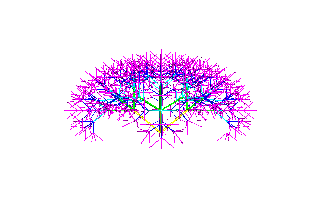5
45
10
A
A = [+cBFA][-cBFA][^cBFA][&cBFA]
B = '(0.7071)
@

Viewing the output shows a 3D tree, but the BASIC form of the fractal is lost. Clearly this is not the way, to
get a good 3D model one should make a so called rotation model of the fractal, i.e. turning it 90 degrees (
in this case!) on its central axis, continuously writing the 2D model, just like rotation models with
mathematical integration. The next file shows the form into four directions. As you can see, I coded the
form twice (definitions A and B), enclosed them within " [ ] ", the startingpoint does not alter, and put
them together just by adding them to each other in the AB command.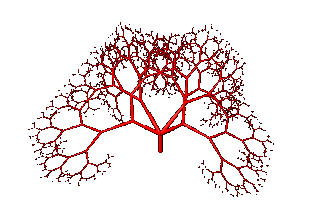9
45
10
AB
A = [F[+FCA][-FCA]]
B = [F[^FCB][&FCB]]
C = '(0.7071)
@

Also possible :)
#--------L-System--C.J.van der Mark-----
#--------Pythagoras semi 3D form--------
5
5
10
Y
Y = X>X
X = A>B>A>B>A>B>A>B>A>B>A>B>A>B>A>B>A>B
A = [F[+(45)FCA][-(45)FCA]]
B = [F[^(45)FCB][&(45)FCB]]
C = '(0.7071)
@Have fun!